## 验证硬实力 硬派SUV哈弗H9冰雪试驾

2018年11月30日 11:59 来源：车行天下 超过：14360次关注

哈弗品牌是国内自主SUV市场的领跑者，而哈弗H9作为哈弗旗下的旗舰车型，25万级的售价直接对垒中高端合资SUV车型，扛起了中国硬派SUV霸主这杆大旗。哈弗H9实力到底有多强?在这个冬天，我们带着哈弗H9来一场冰雪试驾，在冰天雪地中体验它的真正实力。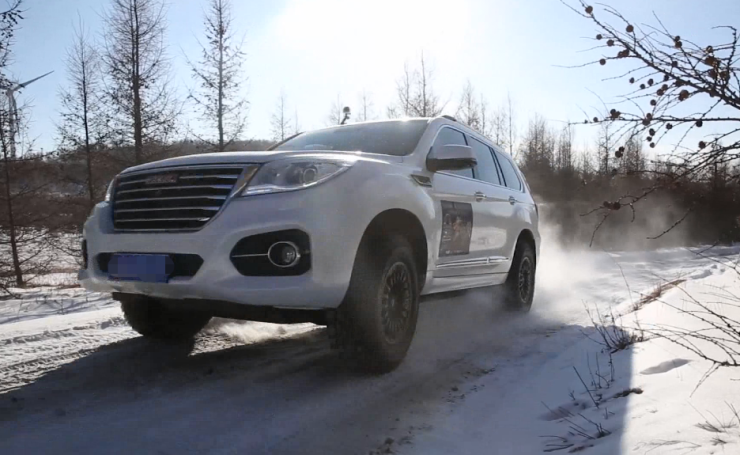我们先从大家都非常关心的动力系统说起。哈弗H9采用的是高功率的2.0L涡轮增压发动机，数据性能非常强大，汽油版最大功率185kW，最大扭矩355Nm;柴油版最大功率140kW，峰值扭矩达到420Nm。实际体验中，凭借涡轮增压的扭矩特性，动力输出还是很给力的。传动系统采用的是ZF的8速手自一体变速器，和动力系统的匹配非常优秀，调校成熟均衡，能够及时领会驾驶者意图，换挡平顺，可以媲美同级合资、甚至是进口产品。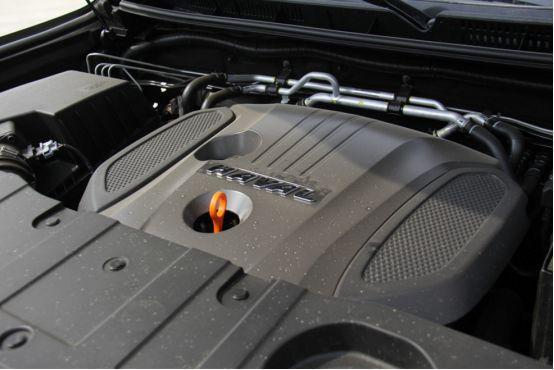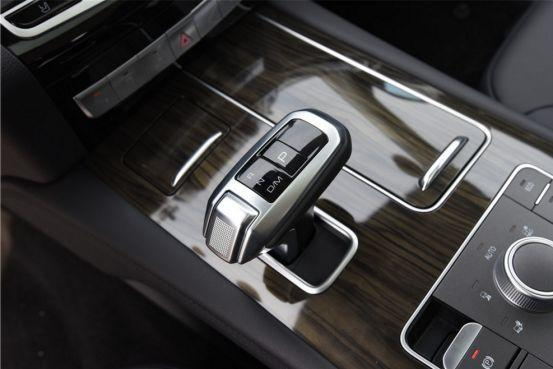底盘构架方面，哈弗H9采用的是非承载式车身，并且采用前双叉臂，后多连杆非独立悬挂的设计，抗扭性能和承载能力一流。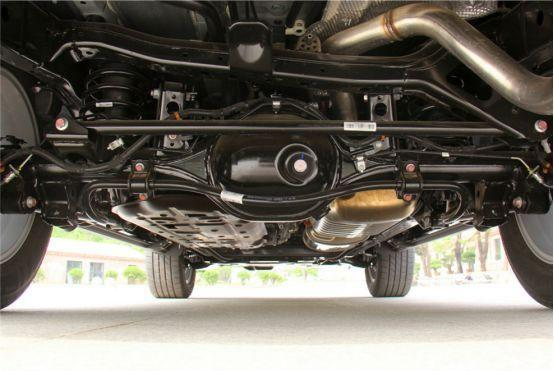哈弗H9的四驱系统采用了来自博格华纳的双速TOD智能适时四驱系统，跟多数适时四驱不同的是，哈弗H9除了有低速四驱扭矩放大(2.48倍)外，后桥中间还有一套伊顿电子限滑差速锁，这是一把集开放、限滑和完全锁止的电子差速锁，能在0.2s内实现完全锁止，而从锁止到放开仅需0.08s，响应灵敏且精准。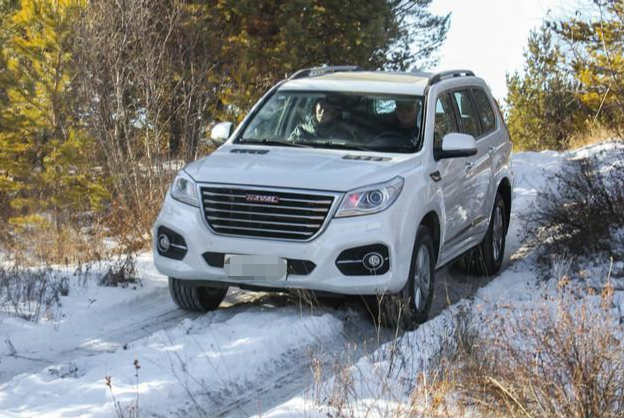哈弗H9的全地形反馈系统大大提升了四驱系统融合度，包含AUTO、运动、沙地、雪地、泥地、ECO以及低速四驱7个模式。不是老司机不要紧，只要你按照当前的路况选择相应的驾驶模式，全地形反馈系统就会帮你控制电子辅助系统的开闭以及动力的输出。自动模式主要是我们日常驾驶的模式，而在冰雪场地，我们则需要切换到雪地模式，系统会适当控制起步时的动力输出，保证行驶平稳。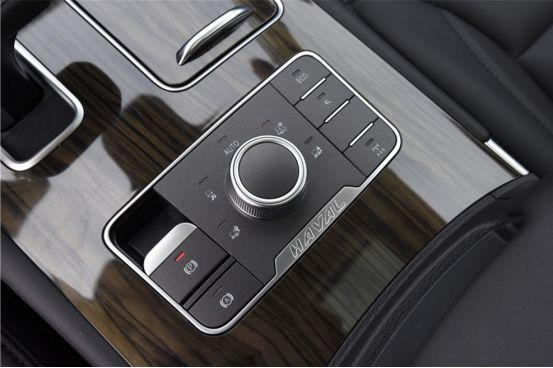介绍了这么多哈弗H9的硬实力，那它在本次冰雪试驾的表现如何呢?

哈弗H9的四驱系统在冰雪路面，响应速度很快，可以及时准确地分配前后轴动力，也时刻保持着可靠稳定的输出，几十公里的中等越野强度路况下，没有出现任何异常或者过热保护。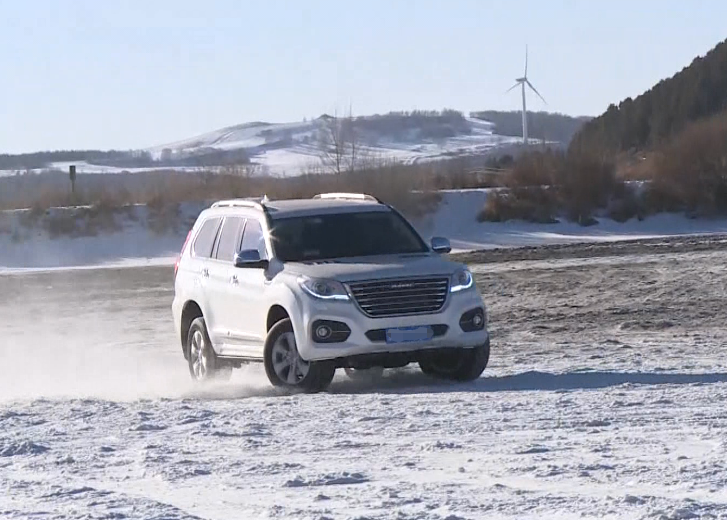哈弗H9的ESP电子系统来自博世，包含ABS车轮防抱死系统、TCS牵引力控制系统以及HDC陡坡缓降系统等。在圆环冰面的试驾，我们可以较为明显地感觉到ESP系统的工作状态，当系统检测到车辆发生侧滑时，系统首先令对应车轮制动，对弯道外侧前轮进行制动并产生一个反作用扭矩使车辆稳定，但如果侧滑趋势没有改善，立刻限制发动机扭矩输出。在此项目中，ESP的一系列干预动作很快速，也对驾驶者挽救车辆有一定的帮助作用。另外，轮胎附着力是一切的基础，所以雪地越野最好提前更换雪地轮胎，保证足够的摩擦力。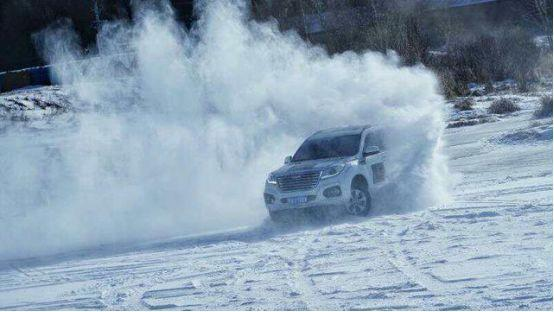这里主要说一下ESP电子系统中的HDC陡坡缓降系统，这是一个很实用的功能，目前很多SUV均有所配备，哈弗H9的陡坡缓降系统默认的陡坡缓降速度是6km/h，实际体验效果很平稳。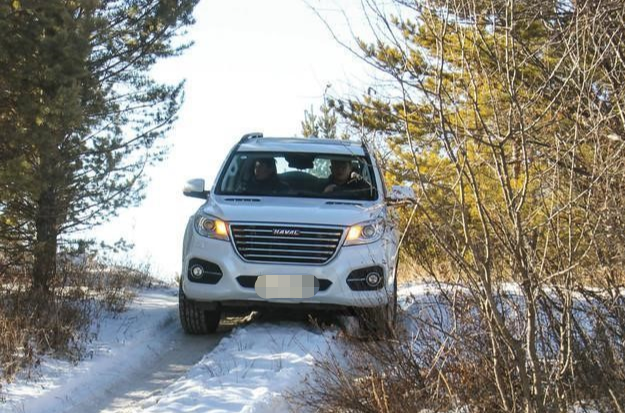而且哈弗H9还可以通过屏幕反馈坡度、倾角、海拔、前后动力分配等信息，很直观也很实用。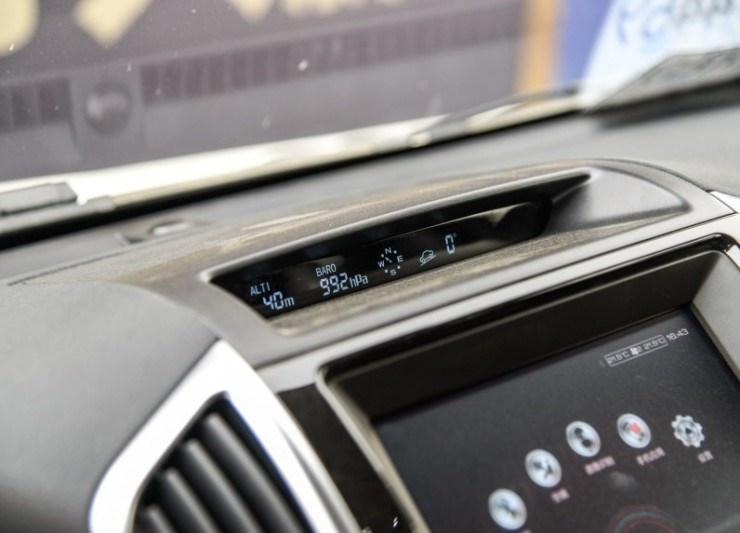在专注越野的同时，哈弗H9还提供了座椅加热功能等舒适性配置，既满足了消费者的越野需求，又保证了舒适的驾乘体验，这也是哈弗H9打动消费者、获得众多消费者拥趸的杀手锏。

在这一场冰雪试驾中，哈弗H9表现突出，四驱系统工作稳定，和动力系统的匹配很协调，反应迅速，提高了行驶的安全性，也能应对中高等强度的越野项目;电子系统很齐备，尤其是全地形控制系统，可以更方便地将车辆调整到最佳的行驶状态;舒适性配置高，充分诠释了高端SUV。小编认为，正是因为哈弗H9有着如此优秀的产品实力，致力于提升用户的出行品质，使其在趋向低迷的SUV市场中仍能保持着自主品牌SUV领头羊的市场地位。

哈弗H9，是一款为越野爱好者而生的车。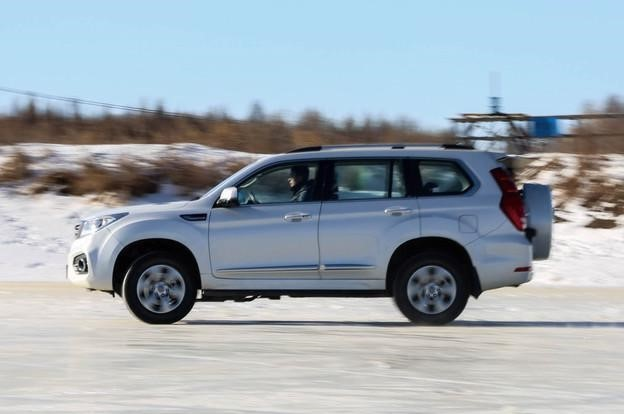#### 相关文章

0-500 字已有评论 0条 查看评论>>

﻿
• 快速找车
• 选择品牌
• 选择品牌
• A  奥迪
• A  阿斯顿·马丁
• A  阿尔法·罗密欧
• B  宝沃
• B  布加迪
• B  巴博斯
• B  保时捷
• B  宾利
• B  奔驰
• B  宝马
• B  本田
• B  别克
• B  标致
• B  比亚迪
• B  宝骏
• B  北汽制造
• B  北汽新能源
• B  北汽幻速
• B  北汽威旺
• B  北京汽车
• B  奔腾
• B  北汽绅宝
• C  长安
• C  长安商用
• C  长城
• C  昌河
• D  大众
• D  道奇
• D  DS
• D  东南
• D  东风风神
• D  东风风行
• D  东风小康
• D  东风风度
• D  东风
• F  福特
• F  丰田
• F  菲亚特
• F  法拉利
• F  福田
• F  福迪
• F  福汽启腾
• G  观致
• G  广汽传祺
• G  广汽吉奥
• G  GMC
• H  红旗
• H  汉腾汽车
• H  哈弗
• H  哈飞
• H  海格
• H  海马
• H  华颂
• H  黄海
• H  华泰
• H  恒天
• J  吉利汽车
• J  捷豹
• J  Jeep
• J  江淮
• J  江铃
• J  金杯
• J  九龙
• J  金旅
• K  凯翼
• K  凯迪拉克
• K  克莱斯勒
• K  科尼塞克
• K  卡威
• K  开瑞
• L  路虎
• L  林肯
• L  劳斯莱斯
• L  兰博基尼
• L  雷克萨斯
• L  铃木
• L  雷诺
• L  理念
• L  力帆
• L  莲花汽车
• L  猎豹
• L  路特斯
• L  陆风
• M  马自达
• M  MG
• M  MINI
• M  玛莎拉蒂
• M  摩根
• M  迈凯轮
• N  纳智捷
• O  欧宝
• O  讴歌
• O  欧朗
• Q  奇瑞
• Q  起亚
• Q  启辰
• R  日产
• R  荣威
• R  瑞麒
• S  三菱
• S  斯威汽车
• S  萨博
• S  smart
• S  斯柯达
• S  斯巴鲁
• S  思铭
• S  双龙
• S  上汽大通
• S  双环
• T  特斯拉
• T  腾势
• W  沃尔沃
• W  五菱汽车
• W  五十铃
• W  威兹曼
• W  威麟
• X  现代
• X  雪佛兰
• X  雪铁龙
• X  西雅特
• Y  一汽
• Y  英菲尼迪
• Y  英致
• Y  依维柯
• Y  野马汽车
• Y  永源
• Z  众泰
• Z  中华
• Z  中兴
• Z  知豆
• 选择车系
• 选择车系
• 车型对比
• 选择品牌
• 选择品牌
• A  奥迪
• A  阿斯顿·马丁
• A  阿尔法·罗密欧
• B  宝沃
• B  布加迪
• B  巴博斯
• B  保时捷
• B  宾利
• B  奔驰
• B  宝马
• B  本田
• B  别克
• B  标致
• B  比亚迪
• B  宝骏
• B  北汽制造
• B  北汽新能源
• B  北汽幻速
• B  北汽威旺
• B  北京汽车
• B  奔腾
• B  北汽绅宝
• C  长安
• C  长安商用
• C  长城
• C  昌河
• D  大众
• D  道奇
• D  DS
• D  东南
• D  东风风神
• D  东风风行
• D  东风小康
• D  东风风度
• D  东风
• F  福特
• F  丰田
• F  菲亚特
• F  法拉利
• F  福田
• F  福迪
• F  福汽启腾
• G  观致
• G  广汽传祺
• G  广汽吉奥
• G  GMC
• H  红旗
• H  汉腾汽车
• H  哈弗
• H  哈飞
• H  海格
• H  海马
• H  华颂
• H  黄海
• H  华泰
• H  恒天
• J  吉利汽车
• J  捷豹
• J  Jeep
• J  江淮
• J  江铃
• J  金杯
• J  九龙
• J  金旅
• K  凯翼
• K  凯迪拉克
• K  克莱斯勒
• K  科尼塞克
• K  卡威
• K  开瑞
• L  路虎
• L  林肯
• L  劳斯莱斯
• L  兰博基尼
• L  雷克萨斯
• L  铃木
• L  雷诺
• L  理念
• L  力帆
• L  莲花汽车
• L  猎豹
• L  路特斯
• L  陆风
• M  马自达
• M  MG
• M  MINI
• M  玛莎拉蒂
• M  摩根
• M  迈凯轮
• N  纳智捷
• O  欧宝
• O  讴歌
• O  欧朗
• Q  奇瑞
• Q  起亚
• Q  启辰
• R  日产
• R  荣威
• R  瑞麒
• S  三菱
• S  斯威汽车
• S  萨博
• S  smart
• S  斯柯达
• S  斯巴鲁
• S  思铭
• S  双龙
• S  上汽大通
• S  双环
• T  特斯拉
• T  腾势
• W  沃尔沃
• W  五菱汽车
• W  五十铃
• W  威兹曼
• W  威麟
• X  现代
• X  雪佛兰
• X  雪铁龙
• X  西雅特
• Y  一汽
• Y  英菲尼迪
• Y  英致
• Y  依维柯
• Y  野马汽车
• Y  永源
• Z  众泰
• Z  中华
• Z  中兴
• Z  知豆
• 选择车系
• 选择车系
• 选择车型
• 选择车型
• 意见反馈